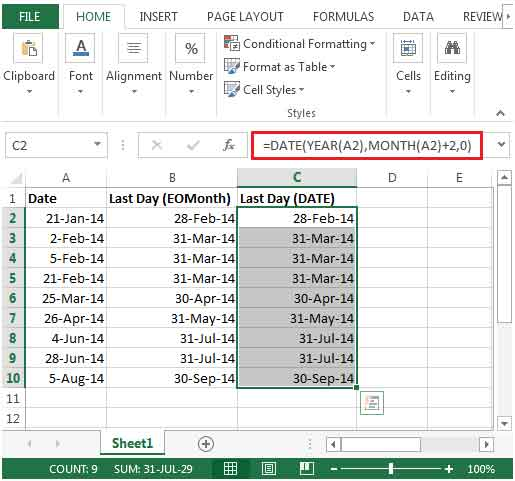# Finding the Last Day of a Given Month in Excel

While preparing tax reports, you may be required to find out the last day of a given month.

Excel has a very useful built in function called EOMONTH. This function is used to calculate the last day of the month. EOMONTH can be used to calculate maturity dates or due dates that take place on the last day of the month.

This function extracts the serial number of the last day of the month before or after a specified number of months.How to calculate date in Excel?

Let us take an example:

We have random dates for year 2014 in column A. In column B, we need to find the last day of the current month using the EOMONTH function. In column C, we need to find the last day of the current month using the DATE function.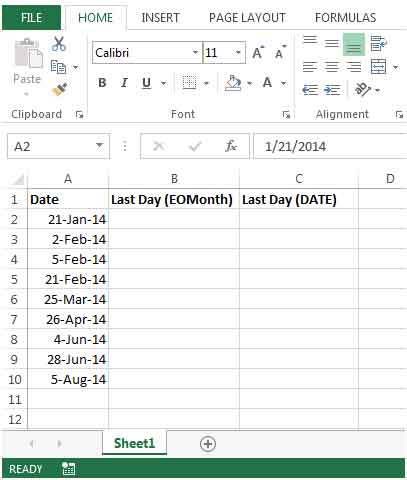• Enter the formula in cell B2 =EOMONTH(A2,0)
• Since we need the last day of the current month, the 2nd parameter in this function will be 0.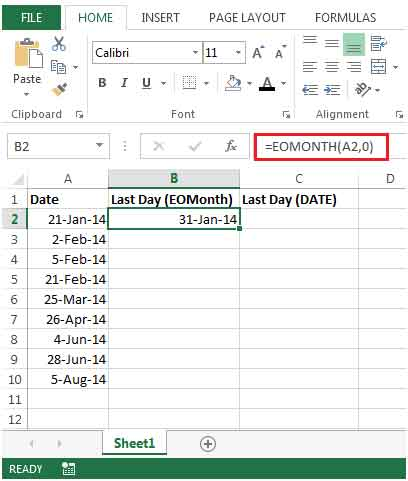• Copy down the formula from cell B2 to the range B3:B10.
• You will get the desired result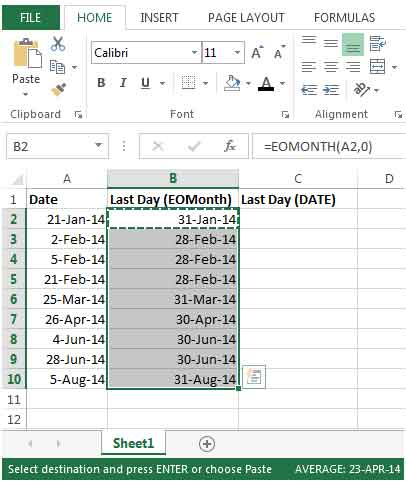• If we need to find the last date of the following month, then we need to change the second argument of the EOMONTH function.  For example –the formula in cell B2 will now be =EOMONTH(A2,1).
• Here, the 2nd parameter is 1, since we need the last date of the following month.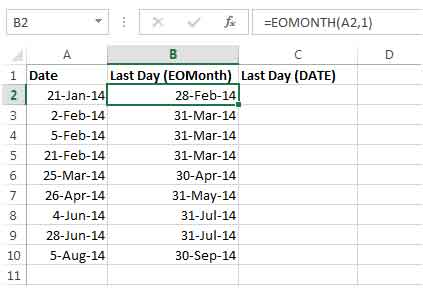This will give us the date for the end of the following month for each date in column A.

We can also use the EOMONTH and TODAYfunctions together to calculate the date at the end of the current month as shown in the following formula:

=EOMONTH(TODAY(),0)

To calculate the date at the end of next month, use the EOMONTH and TODAY functions as shown in the following formula:

=EOMONTH(TODAY(),1)

There is another method to find the last day of the current month, that is by using the DATE function.

• Enter the formula in cell C2 =DATE(YEAR(A2),MONTH(A2)+1,0)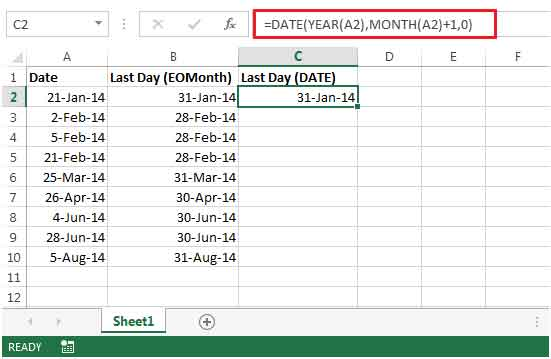• Copy down the formula from cell C2 to the range C3:C10.
• You will get the desired result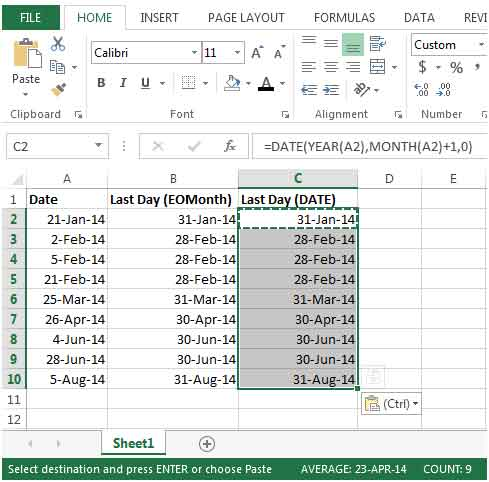• To get the last day of the next month, the formula in cell C2 would be

=DATE(YEAR(A2),MONTH(A2)+2,0)